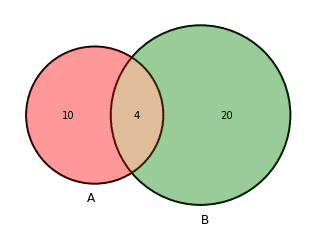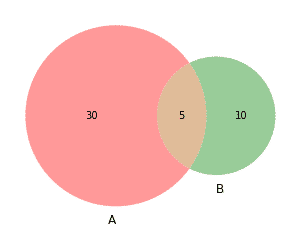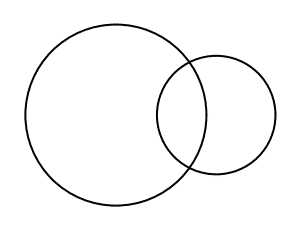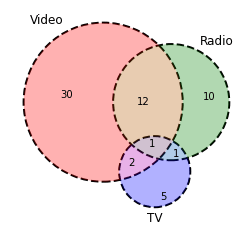# How to Create Venn Diagrams in Python

It’s simple to identify the similarities and contrasts between two or more groups when using a Venn diagram to represent their relationships. In this post, we’ll demonstrate how to make Venn diagrams in Python and how to edit them as needed.There is no out-of-the-box matplotlib or seaborn function that produces a Venn diagram out of the box. So we will used a need library to produce a Venn diagram. This library is called matplotlib-venn so if you haven’t installed this you can easily do this with a pip command

``pip install matplotlib-venn``

Now you are all good with the installation, we can start to produce a variety of different diagrams based on group sizes. We will simply import these from the main library.

``````#import the 2 category Venn diagram plots and visualize the venn2
from matplotlib_venn import venn2
venn2(subsets=(30,10,5)) ``````So you can see that we have defined all the two groups hence the venn2 function. The shared area in the subset parameter of the code is indicated by the the last value in the subset. Now there are different variations like only adding circles.

``````#use only the circles
from matplotlib_venn import venn2_circles
venn2_circles(subsets=(30,10,5)) ``````Now combining these two above graphs will give you something that is a bit more stylish. You can also change the line style parameter to get a slightly different look.

``````#use only the circles and the venn to get a outlined venn diagram
from matplotlib_venn import venn2_circles
venn2_circles(subsets=(10,20,4))
venn2(subsets=(10,20,4)) ``````We can go to a more complex venn3 iteration with will require you show additional connections between major groups. We can alter the alpha, line style, color and labels to fit your specifications.

``````venn3_circles(subsets=(30,10,12,5,2,1,1),linestyle='dashed')• in
• |
• November 20, 2022#### Gaelim Holland

Subscribe
Notify of